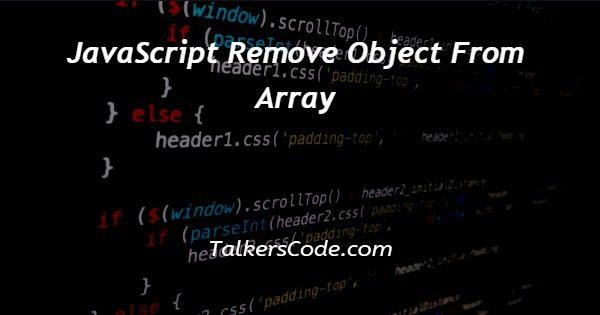# JavaScript Remove Object From Array

Last Updated : Jul 1, 2023In this article we will show you the solution of JavaScript remove object from array, to remove objects from an array we can use various methods.

In this tutorial, we will see different methods to remove objects as,

1. Pop method
2. Shift method
3. Filter method
4. Splice method
5. Arrow method

## Step By Step Guide On JavaScript Remove Object From Array :-

Let us see the step by step guide for understanding these methods,

### Method 1

The first method we will use is the pop method. It is used mostly to remove the last element.

```const array = [1,2,3,4,5]
console.log(array)
array.pop()
console.log(array)```
1. At first create an array.
2. Then display the array using console.log().
3. Using pop function as array.pop() to remove the last element from the array.
4. Using console.log() to display the array after removing.

### Method 2

In the second method, we’re going to use the shift method. It is used mostly to remove the first element.

```const array = [1,2,3,4,5]
console.log(array)
array.shift()
console.log(array)```
1. First create an array.
2. Then display the array using console.log().
3. Using shift function as array.shift() to remove the first element from the array.
4. Using console.log() to display the array after removing.

### Method 3

In the third method, we are going to use the filter method. in this method, we will able to remove the object if the object is present more than once.

```const array = ['a','b','c','d','e','f'];
const newArray = array.filter(( item ) => item !== ' d ')
console.log(newArray)
console.log(array)
```
1. At first create an array
2. Then create a new array
3. Then array.filter() to filter the element to remove.
4. Then to display the new array that consists of elements after filter out the element we want to remove by using console.log() .
5. Also display the original array using console.log().

### Method 4

The fourth method we are going to use splice method.

```const array= ['a','b','c','d','e','f'];
console.log(array)
console.log(array.splice(2,1))
console.log(array)
```
1. At first create an array
2. Using console.log() to display the array
3. Use the splice function by denoting ( index , element position)
4. Then display the array after removing the element.

### Method 5

The last method we are going to use is the arrow method. In this case, we don’t know the position of the object.

```const array1 = ['p','q','r','s','t']
const removeItem = (arr, item) => {
let Array2 = [...arr]
const index = Array2.findIndex((element) => element === item)
if( index!== -1){
Array2.splice(index,1)
return Array2
}
}
console.log(removeItem(array1 , 'r'))
console.log(array1)```
1. First create an array.
2. First finding the position of the element.
3. Then using the splice method
4. Using console.log() to display the array.

## Conclusion :-

Lastly, in conclusion, here we can say that with the help of this article we can remove object from an array using JavaScript.

I hope this article on JavaScript remove object from array helps you and the steps and method mentioned above are easy to follow and implement.